# Design Switching Controllers by Using Simulink Functions

This model shows how you can design switching controllers by combining the power of Stateflow® and Simulink® functions.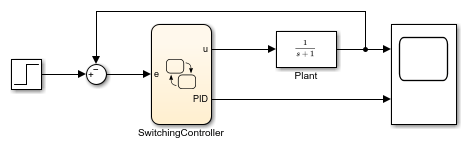The Stateflow chart `SwitchingController` implements a simple switching controller which switches between three states: `STEADY`, `P` and `PID`. When in `STEADY` state, we produce zero control output. When in `P` or `PID`, we delegate to Simulink function call subsystems in order to compute the required control effort.

The Create data for monitoring option for the state `PID` is checked. Therefore, in addition to the control output `u`, the Stateflow chart also produces a logging output with the same name as the state `PID`.

The condition for switching from `P` to `PID` is based on the error being low enough `[e < PID_TRESH]`. `PID_TRESH` is a variable defined in the Model Workspace with a value of 0.3.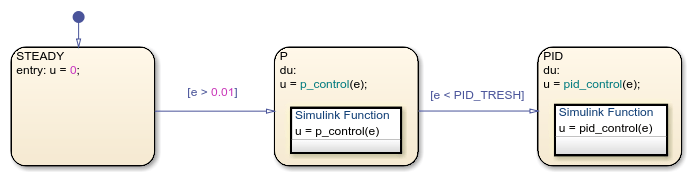The Simulink subsystem `SwitchingController/P.p_control` implements a very simple proportional control with a gain of 3. If we had continued to stay in the state `P`, the steady state gain of the closed-loop system would be 3/4 = 0.75. Therefore, we would get an error of 0.25.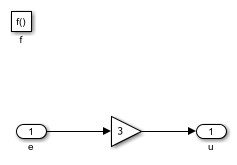The Simulink subsystem `SwitchingController/PID.pid_control` implements a simple PID control strategy. The proportional gain is the same as in `P` thereby ensuring a smooth transition in the control effort.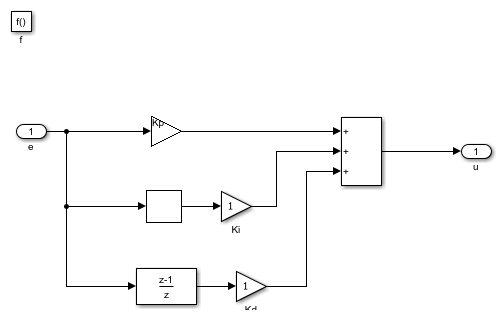When we simulate the model, we notice that the steady state error approaches zero.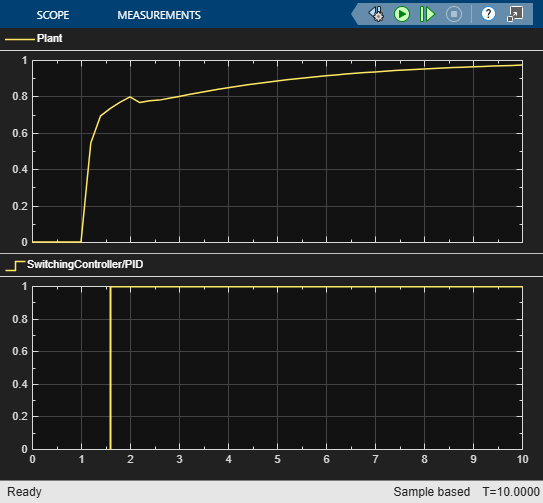In the absence of the PID control, we would have had a steady state error of 0.25. If we change `PID_TRESH` to 0.1, we will never get to `PID`, because the error will never get below 0.25 as long as we are in state `P`.## SupportGet trial now